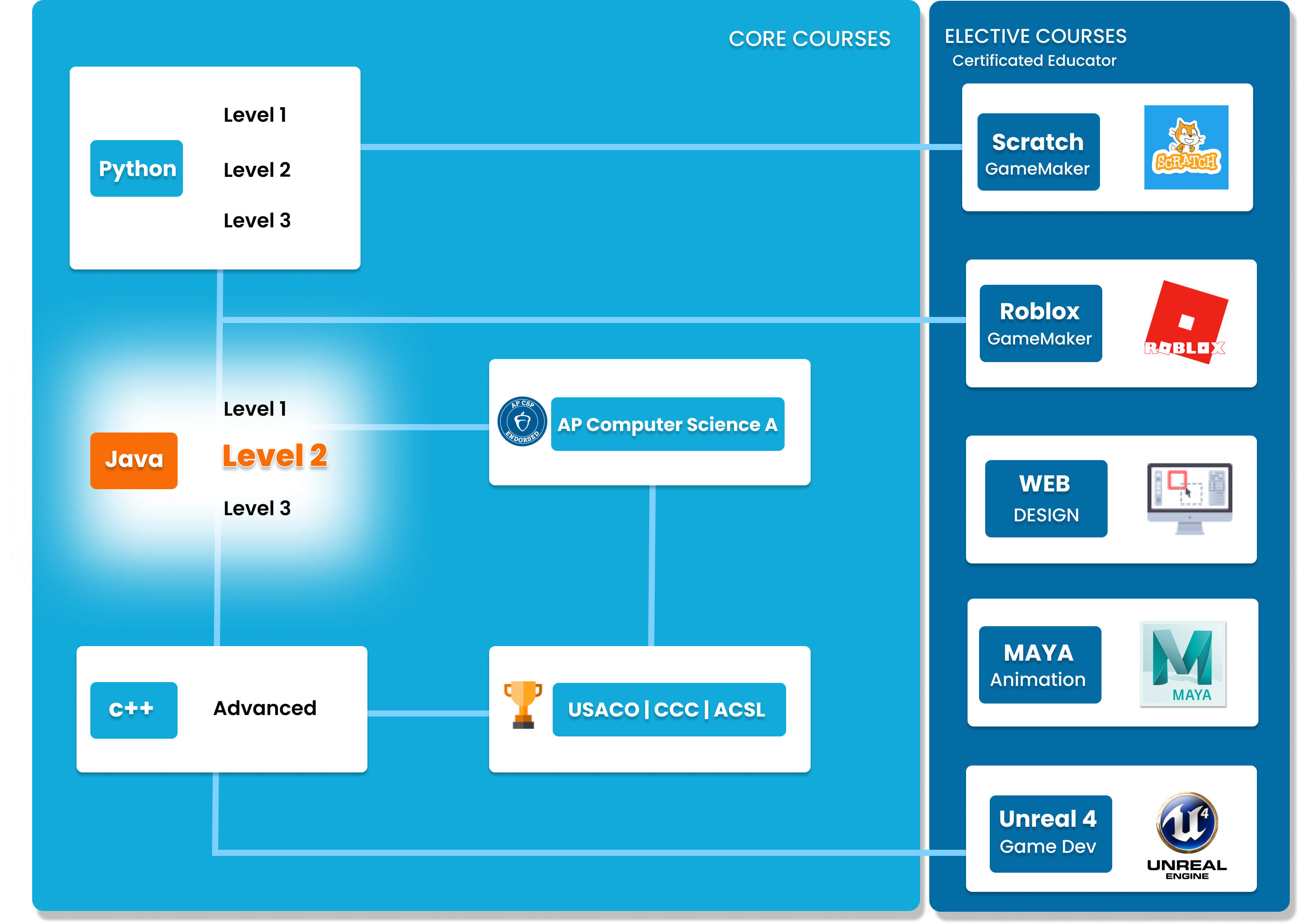# Java Level 2

In this intermediate Java course, students will build on the concepts covered in level 1. It is expected that students have either taken Java level 1, or have a basic understanding of the language. Concepts covered in this course include classes and objects, inheritance and polymorphism, Java standard classes and more. Students will apply these concepts to creating intermediate games, such as Hangman. By the end of this course students will have an intermediate level understanding of Java and will be ready to progress to level 3.##### Who is this course for
Intermediate Students: This course is for students who have completed Level 1 or have previous experience in Java.
This course typically takes 3-6 months to complete. This depends on the student's experience and how fast they can master the concepts and the knowledge. Our instructors move at the pace of the student, it may take extra time for some students to reinforce what they have learned.
##### Curriculum
###### Chapter 1: Classes and ObjectsChapter 1 introduces the fundamental concept of object-oriented programming: Classes and Objects. Students will learn what they are and how they correlate to all things in Java. Additionally, students will learn about access modifiers, methods, scopes, and references.
###### Chapter 2: Inheritance and PolymorphismChapter 2 dives into the topics of inheritance, polymorphism, casting, abstract classes, and interfaces.
###### Chapter 3: Standard ClassesChapter 3 showcases the built-in standard classes in Java, such as the Object, String, Math, and Wrapper Classes.
###### Chapter 4: Program Design and AnalysisChapter 4 dives into fundamental Program Design and Analysis used in the software development industry. Students will learn about topics such as the Waterfall Model, Testing and Debugging, Assertions, and UML Diagrams. All of which are needed for a successful developmental lifecycle.
###### Chapter 5: ReviewChapter 5 is a review of all the previous concepts learned. Students will go through a cumulative review of Levels 1 and 2 through practices questions and mock exams

### You Might Also Be Interested In Our Elective Courses##### Introduction to Number Theory
AGES 10 - 15

This course is designed to build the basics of a complicated mathematical field. It is designed to be a complete introduction to the fundamental concepts of number theory for students in grades 7-10.

The class covers topics such as primes and composites; multiples, divisors, and divisibility; prime factorization and its uses; modular arithmetic and more.##### Introduction to Geometry
AGES 10 - 15

Students in grades 6-10 will build a solid understanding of more advanced geometry concepts in this course. It is designed to expand on the standard high school geometry curriculum and to challenge students with new concepts and problems.

Topics include congruent and similar triangles, circles, 3D geometry, and introductory trigonometry.##### Introduction to Counting and Probability
AGES 10 - 15

To build on the concepts from the Introduction level course, students will build on their Counting and Probability knowledge.

A thorough introduction for students in grades 7-10 to counting and probability topics such as permutations, combinations, Pascal's triangle, geometric probability, basic combinatorial identities, the Binomial Theorem, Pigeonhole Principle Conditional, Probability Graph Theory and more.